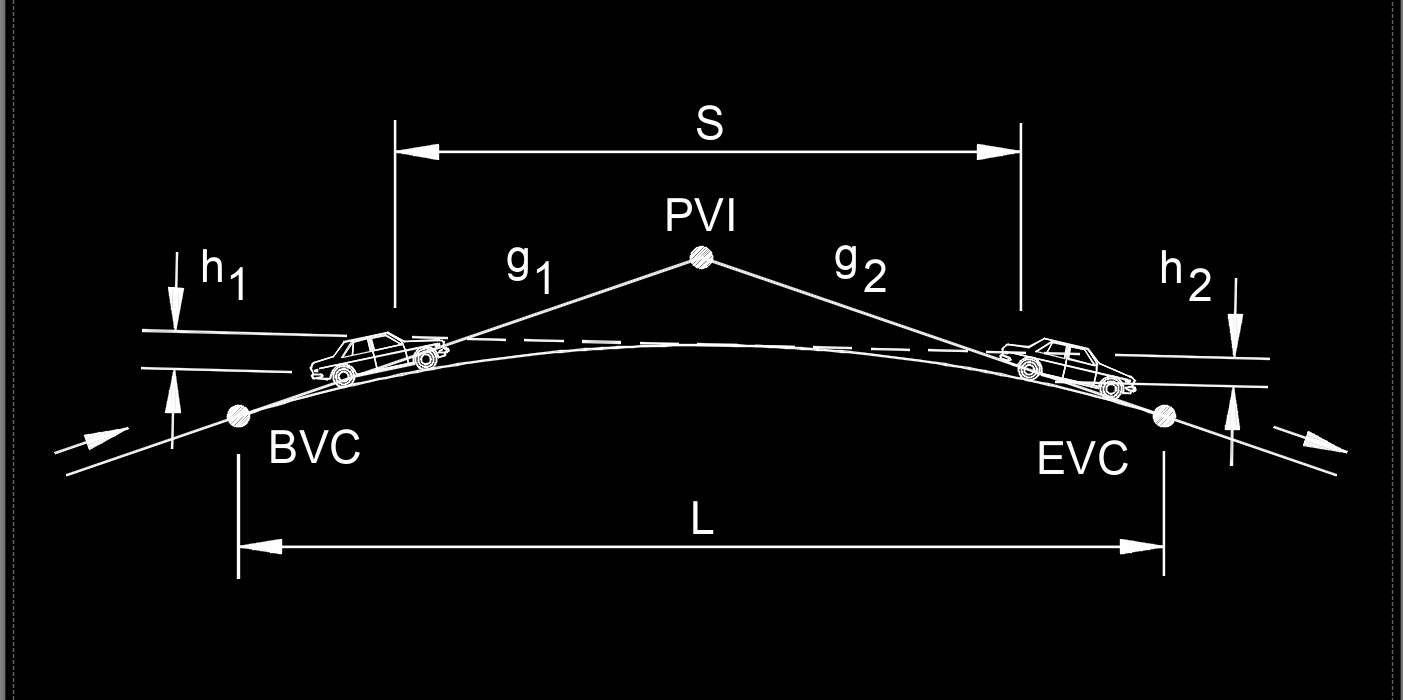Written by Jerry Ratzlaff on . Posted in Civil EngineeringAlgebric difference in grade, abbreviated as A, is the difference in grades.

## Algebric Difference in Grade formula

 $$\large{ A = g_2 \;-\; g_1 }$$

### Where:

$$\large{ A }$$ = algebric difference in grade (%)

$$\large{ g_1 }$$ = tangent grade of a slope given in %

$$\large{ g_2 }$$ = tangent grade of a slope given in %